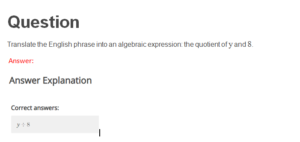# (Answered) MATH114N Week 1 Assignment: Rewrite English Phrases into Algebraic Expressions

Question

Translate the English phrase into an algebraic expression: the quotient of y and 8.

Answer:

# Question

Ruth has pennies and dimes in her pocket. The number of dimes is twelve less than twice the number of pennies. Let p represent the number of pennies. Write an expression for the number of dimes.

Answer:

# Question

The number of students in a school’s Spanish club is ten less than four times the number of students in the chess club. Let c represent the number of students in the chess club. Write an expression for the number of students in the Spanish club.

Answer:

# Question

Translate the English phrase into an algebraic expression: four divided by the sum of x and three.

Answer:

# Question

Translate the English phrase into an algebraic expression: nine times the sum of n and one.

Answer:

# Question

Translate the English phrase into an algebraic expression: the product of 8 and 7.

# Question

Andrea and Helen participated in a donut eating contest. Andrea ate six more than four times the number of donuts that Helen ate. Let d represents the number of donuts Helen ate. Write the expression that gives the number of donuts that Andrea ate.

# Question

Jacqueline and Maria set up bug barns to catch lady bugs. Jacqueline caught ten more than three times the number of lady bugs that Maria caught. If c represents the number of lady bugs Maria caught, write an expression for the number of lady bugs that Jacqueline caught.

# Solution:….Please click the icon below to purchase full solution at \$5